## 22.3 General Root Algebra

This leads us to a discussion of the more general equations for any root.

### A. The General Root Formula, F Series

Let us begin by comparing our equations that we have derived for the F&D series for the square, cube, and fourth roots. Following are the F Series for the square, cube and fourth roots, derived in the preceding pages. F3 is equation 28 under the derivations of the cube roots. F4 is equation 18 under the derivations of the 4th roots. F2 was developed in the previous Notebook.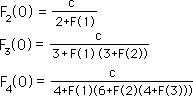The pattern is quite obvious. The coefficients derived from the binomial expansion are rows from Pascal’s triangle, excluding the first ‘one’. If we are trying to find the 4th root, we must include the past 3 expressions when we iterate the series. If we are finding the Nth root we must include the past N-1 expressions in our iteration sequence. Below are two equivalent expressions for the General Root.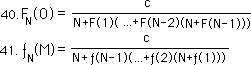The missing coefficients are supplied by the rows from Pascal’s triangle, the normal binomial coefficients. We could supply them but we have a better equation coming just around the corner.

#### Computer testing successful

We have successfully tested this general equation for both 5th and 6th roots. It is impossible to test all possible numbers and all possible roots in this equation because there are infinite amounts of each. However all the numbers tested were direct hits. As before the equation only needed 20 or 30 iterations before surpassing the computer’s accuracy at 14 decimals for the Root Numbers under 10 while it was a bit slower over 10. While we only tested an infinitesimal number of possibilities, the likelihood of an accidental direct hit on even one of these possibilities is so small as to be close to impossible if the equation was wrong. With each successive testing and each direct hit, the infinitesimal possibility is made even smaller.

#### After truth only

Because we are only after the truth, this is sufficient for us. Obviously the truth has not been thoroughly proved mathematically. It has only been demonstrated. Because we are only pretending to be a mathematician, this is good enough for our purposes. It certainly would hold up in a court of law. We are not being arrogant, as much as that we have a lot of material to cover before we die and don’t have the time or mental capacity to spend on the thorough mathematical proofs that scientists require. However this does not distract from the truths that have been discovered. Treat this as science rather than math and it works just fine. Nobody has proved the theory of gravity. It has just been demonstrated successfully an innumerable amount of times.

### B. The General Root Formula, D Series

#### F Series with the circle notation

Now let us look for general patterns in our F Series as a function of the Denominator Series with the circle notation. Each of these equations has been developed in their own sections.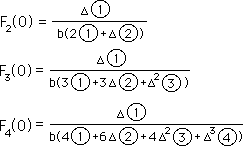#### Numerator Series a function of the past denominator.

The first obvious pattern is that the Numerator Series in each case is a function of the past denominator.We show this generalization below.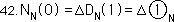#### General Denominator Series for the Nth Root

Listing the Denominator series for square, cube, and 4th roots, the pattern is apparent.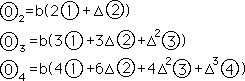Each of the D series has two factors, the first being ‘b’, the denominator of our Root Number. The second factor is similar in some ways to our F Series. The coefficients are the same binomial numbers from Pascal’s triangle minus the first ‘one’. Further the square root D Series is a function of the past two denominators; the cube root D series is a function of the past three denominators; the fourth root D series is a function of the past four denominators. It bears to reason that our Nth D Series would be a function of the past N denominators. The delta coefficients just grow by one with each successive term. Below is the general D series.#### General F Series in Circle Notation

If one looks at the process in which the D2, D3, and D4 series were derived, this pattern is easily seen. Given the General F Series, equations 41 and 42, computer tested but not algebraically proven, let us test the hypothesis that there is a Numerator and Denominator series for the F Series and that the Numerator series equals ∆ multiplied by the past Denominator. This is the same process we employed before. We can express this in the following way.We first substitute this expression into all the F series of Equation 41. Because of the nested nature of the equation the previous denominators cancel out the subsequent numerators as before. Eventually all the denominators except Circle1 cancel out. We multiply top and bottom by this Circle1 and convert to the delta, ∆, terminology and arrive at the following equation, where the coefficients are supplied by Pascal’s triangle as before.#### Computer tested

Not trusting pattern recognition alone, we’ve tested this equation on the computer with 5th and 6th roots, always obtaining the results that we expected. Using the same scientific, not mathematical, reasoning as before, we reason that if we obtain the correct 6th root of 23 from this equation and then obtain the correct 5th root of 105 that while the equation hasn’t been proved, it has been sufficiently demonstrated beyond reasonable doubt. Because we are only after truth, this is fine for us.

#### What are we talking about?

Before getting too far off the track, let us ground ourselves briefly. Our F series is only an approximation of the Root, while our expanding X series is the Root. While the X Series is the Root, a way to approximate its value is through the F Series. This relationship is expressed below again, this time in general form.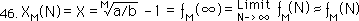This is the ƒ notation system. One can also represent it in the capital F notational system. The capital F notational system is always in the Now. All relations are expressed in terms of the Now. If the number is positive, the event occurred before the Now, i.e. in the past. If the number is negative then it occurred after the Now, i.e. in the future. Thus the infinite limit when it reaches its root is at negative infinity, in the infinite future, using colloquial speech. This is expressed below.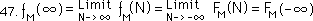#### Reality only an approximation of the ideal

There is one other thing to note. Although the X series is exactly the root, although the infinite limits of the F Series is exactly the root; neither one of these series is real. They both only exist in the imagination, in the idealized reality. The only Series that is real is the F Series and it is only an approximation. Hence making the metaphorical leap: Reality is only an approximation of the ideal. This idea was developed more thoroughly by Plato in his theory of forms.

### C. Fractional Roots

Before simplifying the Root Formula for the F&D Series, let us do the final extension of these series. We started in the prior paper with the F&D Series for the square root of 2. In this paper we have derived and tested F&D Series that can find the Nth Root of any number, when N is an integer. This section will derive an equation, an extension of the Root Formula for the F&D Series, that will compute the fractional roots of any number. While the previous F&D equations could generate integer roots of any number they could not generate fractional roots. This section closes that gap.

#### Proof

We start our proof in the same way that we’ve started every stage of the way. We let (X+1) equal some rational number, a/b, this time raised to the fractional exponent, M/N. In step 2 we raise both sides of the equation to the Nth power. In step 3, as before, we expand the left hand side of the equation in the binomial fashion. In step 4, as always, we subtract 1 from both sides of the equation and factor out an X from what’s left on the left side of the equation. In step 5 we set the constant, c, equal to the more complicated constant on the right hand side of the equation.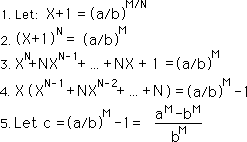#### A More General Expression for ‘c’

Note that the only difference between this more general constant ‘c’ and the constant ‘c’ that we’ve been using is that the ‘a’ and ‘b’ of our original rational number, a/b, are raised to the Mth power. When we were just finding integer roots, M equaled one. Because it had no effect on the equation it was left out all together. Thus this expression for the constant ‘c’ is more general. However when finding integer roots we leave the M out of the expression altogether.

#### General F Series Equation for Fractional Roots

The fact that M can be an integer different than 1 has absolutely no effect upon the left side of the equation. Hence our general Root Equation for fractional roots for the F Series looks identical to that for integer roots. The only difference is that the constant ‘c’ is the more general expression above. This equation is listed below.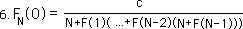#### General D Series Equation for Fractional Roots

Similarly we remember that our F Series can be written as a function of the ratio between consecutive members of the Denominator Series. Again the only difference between the D Series for integer roots and fractional roots are the constants. The form of the equations is identical. Further the constants are not different, just written in a more general form. While the ∆ term for the integer roots equaled a-b, the ∆ term for fractional roots equals a to the M power minus b to the M power. Again when we are speaking of an integer root, M equals one and effectively drops out of the equation.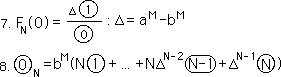Step 8 shows the general expression for the Denominator Series for fractional Roots. Note that the ∆ term is in the more general sense listed in Step 7 and that the coefficient b is now raised to the M power. Otherwise it is an identical expression to the General D Series for integer Roots. Thus without great fanfare we have written a general expression for the F&D Series for the fractional Root, M/N, of any positive rational number, a/b.